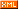# zero_learner

:: :: :: :: :: :::: ::9 随笔 :: 1 文章 :: 80 评论 :: 0 引用

import numpy as np

def bin(bins):
assert isinstance(bins, (list, tuple))
def scatter(x):
if x == 0: return 0
for i in range(len(bins)):
if x <= bins[i]: return i + 1
return len(bins)
return np.frompyfunc(scatter, 1, 1)

# descrete
o2o_result_num = data[:,0]
o2o_has_result = o2o_result_num[o2o_result_num > 0]
bins = [ np.percentile(o2o_has_result, x) for x in range(10, 101, 10) ]
data[:,0] = bin(bins)(o2o_result_num)

 1 from sklearn import cross_validation
2 from sklearn import tree
3 from sklearn import ensemble
4 from sklearn import linear_model
5 from sklearn import svm
6
7 lr = linear_model.LogisticRegression()
8 lr_scores = cross_validation.cross_val_score(lr, train_data, train_target, cv=5)
9 print("logistic regression accuracy:")
10 print(lr_scores)
11
12 clf = tree.DecisionTreeClassifier(criterion='entropy', max_depth=8, min_samples_split=5)
13 clf_scores = cross_validation.cross_val_score(clf, train_data, train_target, cv=5)
14 print("decision tree accuracy:")
15 print(clf_scores)
16
17 rfc = ensemble.RandomForestClassifier(criterion='entropy', n_estimators=3, max_features=0.5, min_samples_split=5)
18 rfc_scores = cross_validation.cross_val_score(rfc, train_data, train_target, cv=5)
19 print("random forest accuracy:")
20 print(rfc_scores)
21
22 etc = ensemble.ExtraTreesClassifier(criterion='entropy', n_estimators=3, max_features=0.6, min_samples_split=5)
23 etc_scores = cross_validation.cross_val_score(etc, train_data, train_target, cv=5)
24 print("extra trees accuracy:")
25 print(etc_scores)
26
28 gbc_scores = cross_validation.cross_val_score(gbc, train_data, train_target, cv=5)
30 print(gbc_scores)
31
32 svc = svm.SVC()
33 svc_scores = cross_validation.cross_val_score(svc, train_data, train_target, cv=5)
34 print("svm classifier accuracy:")
35 print(svc_scores)

 1 logistic regression accuracy:
2 [ 0.76953125  0.83921569  0.85433071  0.81102362  0.83858268]
3 decision tree accuracy:
4 [ 0.73828125  0.8         0.77559055  0.71653543  0.83464567]
5 random forest accuracy:
6 [ 0.75        0.76862745  0.76377953  0.77165354  0.80314961]
7 extra trees accuracy:
8 [ 0.734375    0.78039216  0.7992126   0.76377953  0.79527559]
10 [ 0.7578125   0.81960784  0.83464567  0.80708661  0.84251969]
11 svm classifier accuracy:
12 [ 0.703125    0.78431373  0.77952756  0.77952756  0.80708661]

lr = lr.fit(train_data, train_target)

1 from sklearn.externals import joblib
2 joblib.dump(lr, 'D:\lr.model')
3 import pickle
4 bin_file = open(r'D:\result_bin.data', 'wb')
5 pickle.dump(bins, bin_file)
6 bin_file.close()

 1 # load result bin data and model
2 bin_file = open(r'D:\result_bin.data', 'rb')
4 bin_file.close()
5
7
9 query = np.genfromtxt(r'D:\o2o_query_rec\all_query', dtype='U2', comments=None, converters={0: lambda x: str(x, 'utf-8')})
10 feature = np.loadtxt(r'D:\o2o_query_rec\all_features', dtype='int', delimiter='\001')
11
12 # descrite
13 feature[:,0] = bin(bins)(feature[:,0])
14 feature[:,1] = ufunc_segment(feature[:,1])
15
16 # predict
17 result = lr.predict(feature)
18
19 # save result
20 #np.savetxt(r'D:\o2o_query_rec\classify_result.txt', np.c_[query, result], fmt=u"%s", delimiter="\t")
21 result_file = open(r'D:\o2o_query_rec\classify_result.txt', 'w')
22 i = 0
23 for q in query:
24     result_file.write('%s\t%d\n' % (q, result[i]))
25     i += 1
26 result_file.close()

posted on 2014-05-24 20:32 zero_learner 阅读(...) 评论(...) 编辑 收藏